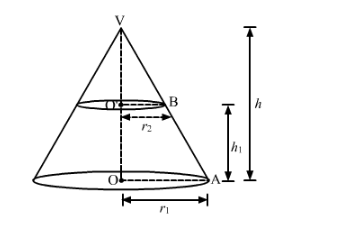# If r1 and r2 denote the radii of the circular bases`
Question:

If r1 and r2 denote the radii of the circular bases of the frustum of a cone such that r1 > r2, then write the ratio of the height of the cone of which the frustum is a part to the height fo the frustum.

Solution:Since, $\Delta V O^{\prime} B \sim \Delta V O A$

Therefore,

In $\Delta V O^{\prime} B-\Delta V O A$

$\frac{O^{\prime} B}{O A}=\frac{O^{\prime} V}{O V}$

$\frac{r_{2}}{r_{1}}=\frac{h-h_{1}}{h}$

$\frac{r_{2}}{r_{1}}=1-\frac{h_{1}}{h}$

$\frac{h_{1}}{h}=1-\frac{r_{2}}{r_{1}}$

$=\frac{r_{1}-r_{2}}{r_{1}}$

Hence,

The ratio of the height of cone of which the frustum is a part to the height fo the frustum.

$\frac{O V}{O O^{\prime}}=\frac{h}{h_{1}}=\frac{r_{1}}{r_{1}-r_{2}}$

Hence, $h: h_{1}=r_{1}:\left(r_{1}-r_{2}\right)$